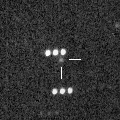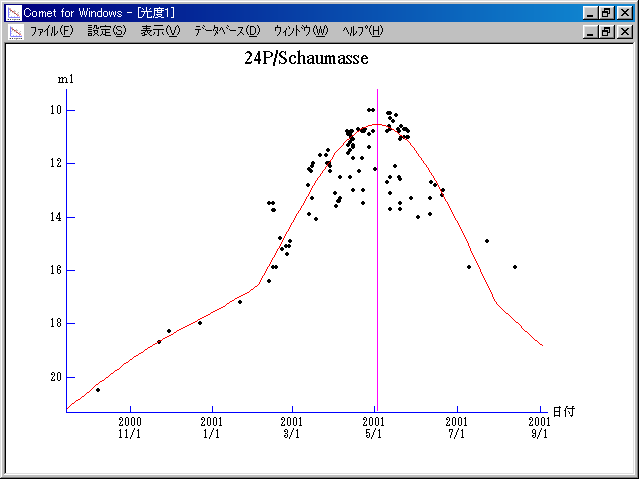# \$B%7%g!<%^%9WB@1(B

24P/Schaumasse (2001)

 English version Home page Updated on December 31, 2013###\$B###\$B50F;MWAG(B

```   The following improved orbital elements by Kenji Muraoka,
are from 523 observations 1984 to 2001, including 9 Planets,
Moon and 5 minor planets perturbations and non-gravitational
effect of style II.  The mean residual is +/- 0.80 arc seconds.

Epoch  =  2001 May  11.0  TT       JDT = 2452040.5
T  =  2001 May   2.65966       +/- 0.00017 (m.e.) TT
Peri. =   57.87344                +/- 0.00010
Node  =   79.83119                +/- 0.00003   (2000.0)
Incl. =   11.75149                +/- 0.00001
q  =    1.2050046              +/- 0.0000004 AU
e  =    0.7048079              +/- 0.0000001
a  =    4.0821028              +/- 0.0000004 AU
ns =    0.11950283             +/- 0.00000002
P  =    8.248                  +/- 0.0000013  years
A1  =   +0.126                  +/- 0.014
A2  =   -0.05861                +/- 0.00007
```

###\$B@1?^(B###\$B8wEYJQ2=(B

```        m1 = 12.0 + 5 log\$B&\$(B + 17.0 log r  [   ,-90]  (              \$B!A(B2001\$BG/(B02\$B7n(B01\$BF|(B)
m1 =  6.0 + 5 log\$B&\$(B + 45   log r  [-90, 90]  (2001\$BG/(B02\$B7n(B01\$BF|!A(B2001\$BG/(B07\$B7n(B31\$BF|(B)
m1 = 12.0 + 5 log\$B&\$(B + 17.0 log r  [ 90,   ]  (2001\$BG/(B07\$B7n(B31\$BF|!A(B              )
```##### \$B50F;MWAG\$OB<2,7r<#;a\$N7W;;\$K\$h\$k\$b\$N\$G\$9!#(B \$B@1?^\$O(B StellaNavigator Ver.2.0 for Windows (\$B%"%9%H%m%"!<%D(B \$BJTCx(B / \$B%"%9%-!<=PHG6I4)(B) \$B\$G:n@.\$7\$?\$b\$N\$G\$9!#(B \$B8wEY%0%i%U\$O(BComet for Windows\$B\$G:n@.\$7\$?\$b\$N\$G\$9!#(BCopyright(C) Seiichi Yoshida (comet@aerith.net). All rights reserved.